Spitzer Documentation & Tools
IRAC Instrument Handbook

### 5.1.4        insbposdom (InSbĀarray sign flipping)

The IRAC InSbĀarrays (channels 1 and 2) were operated in such a way that flux appeared negative in the raw data (Figure 5.2). That is, data numbers start at 65,535 (16-bit max) for zero light levels and became increasingly close to 0 as light levels increased. The INSBPOSDOM module rectified this so that increasing DNĀ(ōdata number,ö denotes the pixel value, similar to analog-to-digital unit ADU often used in optical data) yielded increasing flux (0 to 65,535), as is more common. This was done by

 Aout = (65,535 - Ain) (5.3)

where A is the pixel intensity in DNĀfor the two InSbĀarrays (ACHANID = 1 or 2). ACHANID was turned into CHNLNUM in the BCDĀheaderĀby the last step in the pipeline.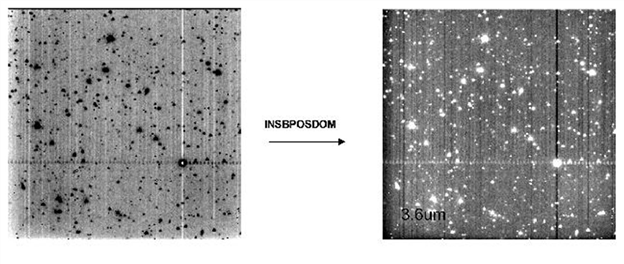Figure 5.2: Effect of insbposdom . insbposdom worked only on the two InSbĀarrays (channels 1 & 2) and reversed the sense of intensities. Left is before insbposdom ran and right is after.

### 5.1.5        cvti2r4 (byte type changing)

Data were converted from the native unsigned 16-bit format used by IRAC to the 32-bit floating point format used in astronomical calculations. At this point, the following DNĀwas added to all the pixels in order to account for the bias introduced by the spacecraft on-board bit truncation.

-0.5 x (1 - 2-ABARREL) for channels 1 and 2

+0.5 x (1 - 2-ABARREL) for channels 3 and 4

Here, ABARREL is the barrel shiftĀnumber keyword where the bit truncation occurred (see Section 5.1.7). Also, if the headerĀindicated that any rows or columnsĀwere blank (usually due to data loss during transmission from Spitzer to the ground), then those pixels were set equal to NaN (Not a Number).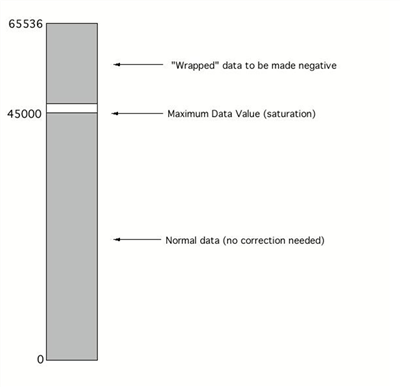Figure 5.3: Diagram of the wrapping of negative values due to truncation of the sign bit.

### 5.1.6        Wraparound Correction: iracwrapdet and iracwrapcorr

IRAC raw data sometimes required correction for wraparound, wherein DNĀvalues were actually multi-valued. That is, a given DN actually corresponded to more than one possible flux level.

iracwrapdet (sign truncation wraparound)

As a means of data compression, IRAC discarded the sign bit of its data before transmission to the ground. IRAC used the 2s-complement storage system for negative numbers. In this system, negative numbers were coded without a negative sign, but with the bits flipped and 1 added. For example, with 16 bits, ¢1 is represented by 65535 in unsigned integer form. However, this created an ambiguity in that negative values appeared in the raw data as very large positive numbers. By design, the detector reached physical saturationĀbefore ōelectronicö (A/D) saturation (Figure 5.3). The maximum physical values the detectors reached were around 45,000 DNĀfor the InSbĀarrays and 55,000 DNĀfor the Si:AsĀarrays, which were less than the maximum 16-bit value of 65,535. Values above these limits should be wrapped negative values. This module then flagged any pixels lying in the wraparound DN region. If any part of the image was near the saturation value (typically either 45,000 DN in channels 1 and 2 or 55,000 DN in channels 3 and 4, in cryogenic mission) then surrounding pixels may have been near saturation.

iracwrapcorr (wraparound correction)

This module used the flag bits set by the previous module and attempted to correct the wraparound problem (Figure 5.4). Note that both the sign truncation and the non-linearityĀwraparound, i.e., doughnuts (see discussion on saturationĀin Section 7.2.1), were corrected in the pipeline. The sign truncation correction was simply

 Acorrected = Auncorrected - 65535. (5.4)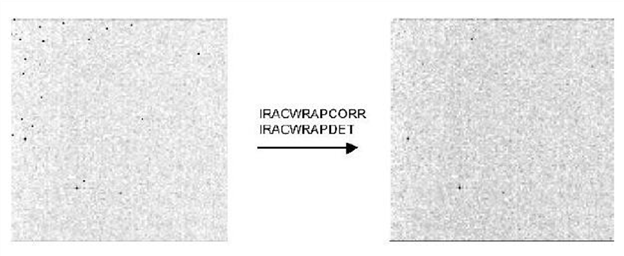Figure 5.4: Application of iracwrapcorr to channelĀ1 data. The many apparently hot pixelsĀwere actually wrapped negative values, which were detected based on their data values vastly exceeding the physical saturationĀvalue for the detectors, and corrected by subtracting the appropriate value. Real hot pixels did not exceed the physical saturation value, and hence were not changed.

### 5.1.7        iracnorm (FowlerĀsampling renormalization)

IRAC data were taken with FowlerĀ(multi) sampling in order to reduce read noise. This was done by non-destructively reading the array multiple times (set by the FowlerĀnumber), and accumulating the sum into an internal register. Since these reads were summed, the result had to be divided by the number of reads in order to get the actual number of DN. Additionally, when data were transmitted to the ground, a variable number of least significant bits were discarded as a means of data compression (Figure 5.5).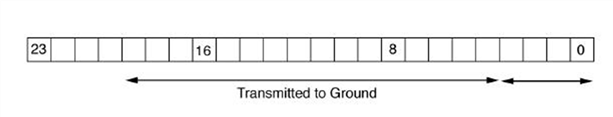Figure 5.5: Illustration of bit truncation. Bit truncation was used by IRAC for ground transmission, necessitating iracnorm. The internally stored 24-bit word was truncated to 16 bits, with a sliding window set by the barrel shiftĀvalue. The figure illustrates the ABARREL=4 case.

In order to correct for the effects of bit-truncation and FowlerĀsampling the data were transformed by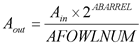. (5.5)

Note that in normal usage the FowlerĀnumber and barrel shift actually used and commanded by the SSC were such that they canceled, i.e.,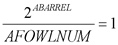(5.6)

and hence observers should not be surprised if this module normally appeared to do nothing.

### 5.1.8        snestimator (initial estimate of uncertainty)

The module snestimator calculated the uncertainty of each pixel based on the input image (here, the input image was the output of iracnorm). The uncertainty for each pixel was estimated as the Poisson noise in electrons, and the read noise was added in quadrature. The formula for the calculation was as follows: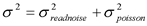(5.7)

To obtain an expression in DN, σ Āwas divided by gain. This uncertainty imageĀwas carried through the pipeline, and additional uncertainties (e.g., darkĀand flatĀuncertainties) were added in quadrature, when appropriate.